# CAT 2019 Question Paper | Quants Slot 1

###### CAT Previous Year Paper | CAT Quants Questions | Question 24

Once in a while in CAT 2019, there were questions that really looked scary. This is one such question which has a couple of algebraic concepts rolled into it. Try taking time out to solve these kind of questions as  part of your CAT Preparation. Practicing more such questions from CAT previous year paper will be most helpful to master such tough questions

Question 24 : If m and n are integers such that ($$sqrt{2}$)19 34 42 9m 8n = 3n 16m$∜64) then m is

1. -16
2. -24
3. -12
4. -20

## Best CAT Coaching in Chennai

#### CAT Coaching in Chennai - CAT 2022Limited Seats Available - Register Now!

This question can be solved by comparing powers of 2 and 3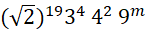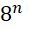=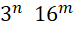(∜64)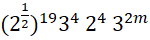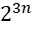=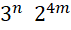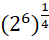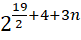.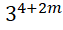=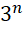.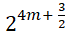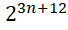.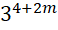=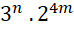Comparing the powers in both sides,
3n + 12 = 4m
4 + 2m = n
Substituting the value of n we get,
3 (4 + 2m) + 12 = 4m
12 + 6m + 12 = 4m
24 = -2m
m = -12

The question is "If m and n are integers such that ($$sqrt{2}$)19 34 42 9m 8n = 3n 16m$∜64) then m is "

##### Hence, the answer is -12

Choice C is the correct answer.

###### CAT Coaching in ChennaiCAT 2023

Classroom Batches Starting Now! @Gopalapuram

###### Best CAT Coaching in Chennai Introductory offer of 5000/-

Attend a Demo Class

##### Where is 2IIM located?

2IIM Online CAT Coaching
A Fermat Education Initiative,
58/16, Indira Gandhi Street,
Kaveri Rangan Nagar, Saligramam, Chennai 600 093

##### How to reach 2IIM?

Mobile: (91) 99626 48484 / 94459 38484
WhatsApp: WhatsApp Now
Email: info@2iim.com